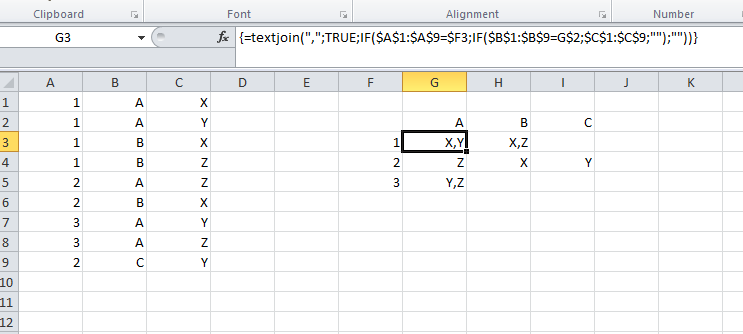2 views
in BI

Given a list of triples I want to get a relationship matrix as follows.

1 A X

1 A Y

1 B X             A   B   C

1 B Z         1  X,Y X,Z

2 A Z   ==>   2   Z   X   Y

2 B X         3   Y   Z

3 A Y

3 A Z

2 C Y

(How) can this be done in Excel/VB/PowerBI or similar?

by (47.2k points)
• You can generate an array which will hold either the value of the third column or a blank depending on whether the value should be included using an array formula. You can then join them using TEXTJOIN.  As TEXTJOIN is new in Office 365, if you don't have it you'll first need to define it as a function this way.

Function TEXTJOIN(Delimiter As String, IgnoreBlanks As Boolean, ParamArray Text() As Variant) As String

Dim Item As Variant, V As Variant, Arr As Variant

For Each Item In Text

If VarType(Item) > 8191 Then

For Each V In Item

If Len(V) > 0 Or (Len(V) = 0 And Not IgnoreBlanks) Then TEXTJOIN = TEXTJOIN & Delimiter & V

Next

Else

TEXTJOIN = TEXTJOIN & Delimiter & Item

End If

Next

TEXTJOIN = Mid(TEXTJOIN, Len(Delimiter) + 1)

End Function

• Now back to your problem, assuming your data is in A1:C9 and the table you want is at F2:I5 (with line 2 and column F containing the indexes) you'll need to use this formula in G3: {=TEXTJOIN(",";TRUE;IF(\$A\$1:\$A\$9=\$F3;IF(\$B\$1:\$B\$9=G\$2;\$C\$1:\$C\$9;"");""))}:• If you're unfamiliar with array formulas, please note that you'll need to use CTRL+SHIFT+ENTER to enter it. You'll then need to copy G3 and paste it to the other cells of your table (excel will be finicky about that if you try to paste to a range including the cell you copied an array formula from, so you might have to do it in several pastes); and the formula will use the proper indexes as \$F3 and G\$2 are relative.